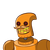# If α, β are the zeros of the polynomial f(x) = x2 + x + 1, then 1 /a + 1 /b= …….a) 1 b)

If α, β are the zeros of the polynomial f(x) = x2 + x + 1, then 1
/a
+
1
/b= …….

a) 1
b) -1
c) 0
d) None of these​

### 1 thought on “If α, β are the zeros of the polynomial f(x) = x2 + x + 1, then 1<br /> /a <br /> +<br /> 1<br /> /b= …….<br /><br />a) 1 <br />b)”

1.Step-by-step explanation:

Given $$\alpha and\beta$$ are the roots of Polynomial f(x)=x^2+x+1   where  (a=1 , b=1 , c=1)

We have To find 1/$$\alpha$$ + 1/$$\beta$$=$$(\alpha +\beta )/\alpha \beta$$     . . . ..(1)

And we also know that sum of roots(zeroes) of quadratic polynomial = -b/a

$$\alpha +\beta =-b/a$$=-1/1=-1  . . . .. (2)

Product of roots of quadratic polynomial =c/a

$$\alpha \beta =c/a=1/1=1$$   . . . . . .(3)

Put the values of (2) and (3) in (1)

we get

-1/1=-1 ANS